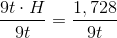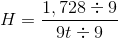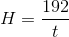# ISEE Upper Level Quantitative : How to find the length of an edge

## Example Questions

### Example Question #2 : Solid Geometry

A cube has sidelength one and one-half feet; a rectangular prism of equal volume has length 27 inches and height 9 inches. Give the width of the prism in inches.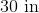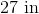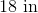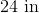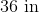Explanation:

One and one half feet is equal to eighteen inches, so the volume of the cube, in cubic inches, is the cube of this, or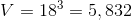cubic inches.

The volume of a rectangular prism is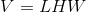Since its volume is the same as that of the cube, and its length and height are 27 and 9 inches, respectively,  we can rewrite this as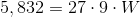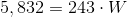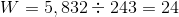The width is 24 inches.

### Example Question #3 : Solid Geometry

A cube has sidelength one and one-half feet; a rectangular prism of equal surface area has length 27 inches and height 9 inches. Give the width of the prism in inches.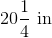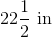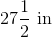Explanation:

One and one half feet is equal to eighteen inches, so the surface area of the cube, in square inches, is six times the square of this, or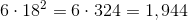square inches.

The surface area of a rectangular prism is determined by the formula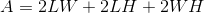.

So, with substitutiton, we can find the width: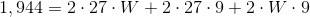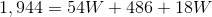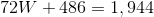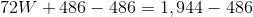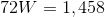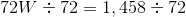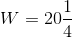inches

### Example Question #4 : Solid Geometry

A rectangular prism has volume one cubic foot; its length and width are, respectively, 9 inches andinches. Which of the following represents the height of the prism in inches?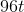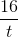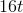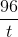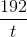Explanation:

The volume of a rectangular prism is the product of its length, its width, and its height. The prism's volume of one cubic foot is equal to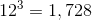cubic inches.

Therefore,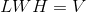can be rewritten as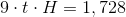.

We can solve for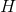as follows: